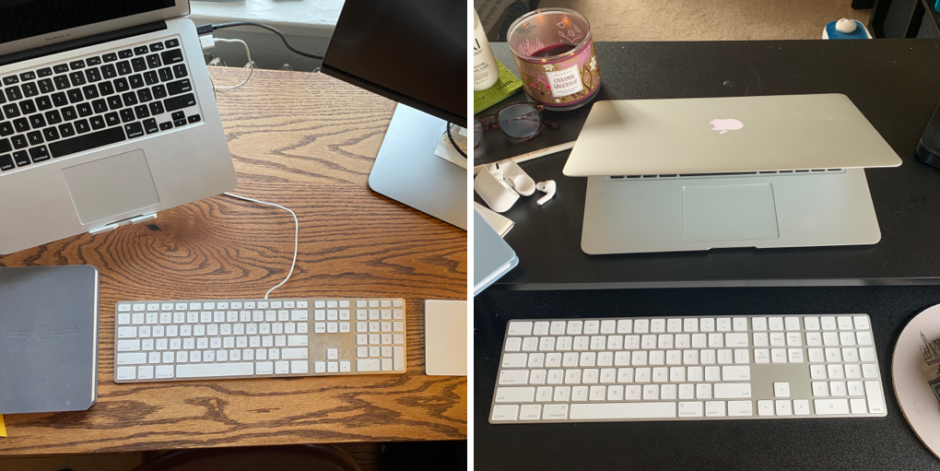Fuzzy Math Spotlight: Lily & Abbie

Posted by Fuzzy Math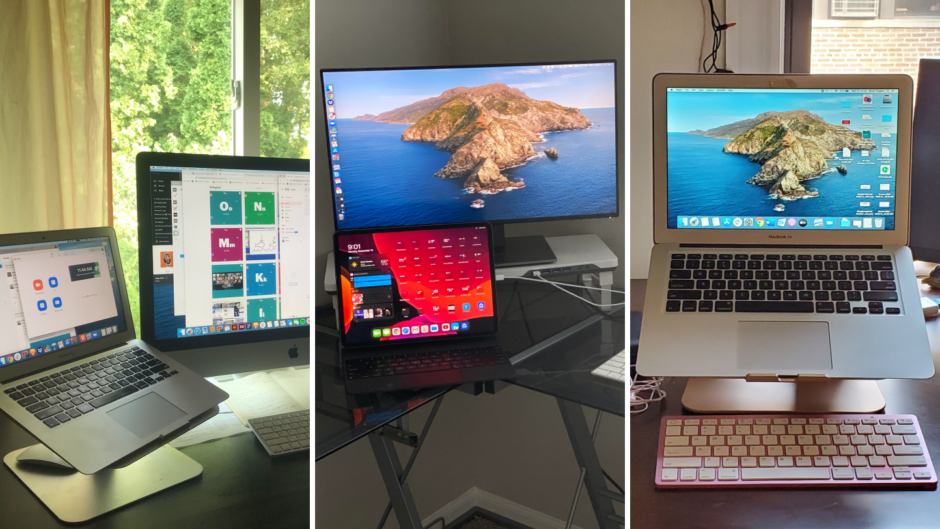Fuzzy Math Spotlight: Bert, Alfreda & Raven

Posted by Fuzzy Math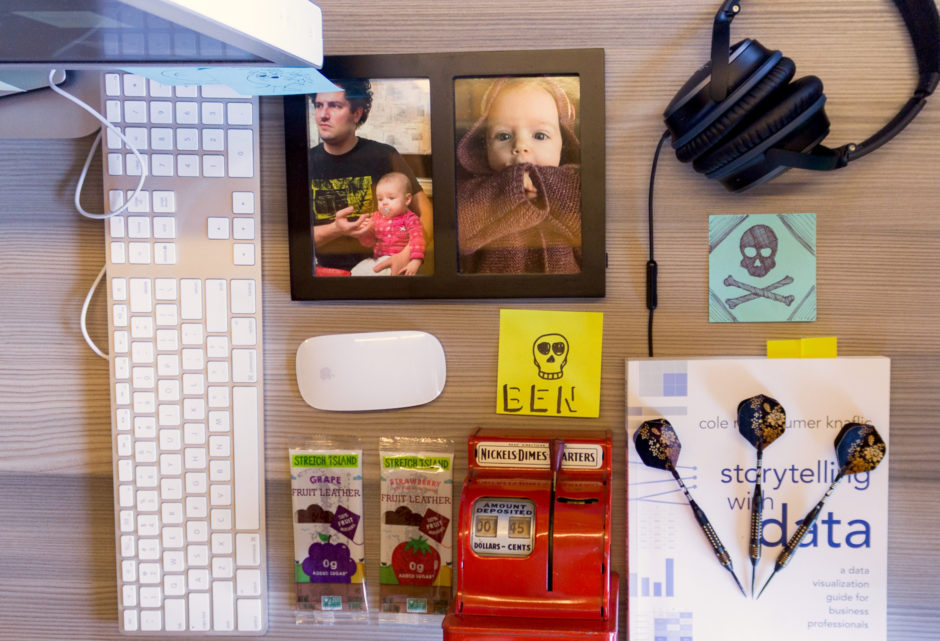Fuzzy Math Spotlight: Ben Ihnchak

Posted by Fuzzy MathFuzzy Math Spotlight: Mark Baldino

Posted by Fuzzy Math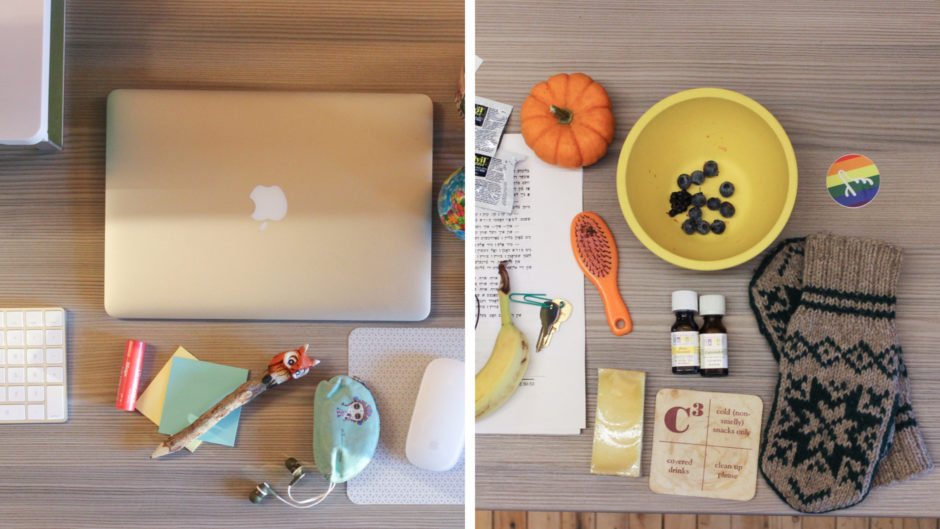Fuzzy Math Spotlight: Ana & Rachel Schastok

Posted by Fuzzy MathFuzzy Math Spotlight: Rachel V. & Nick

Posted by Fuzzy Math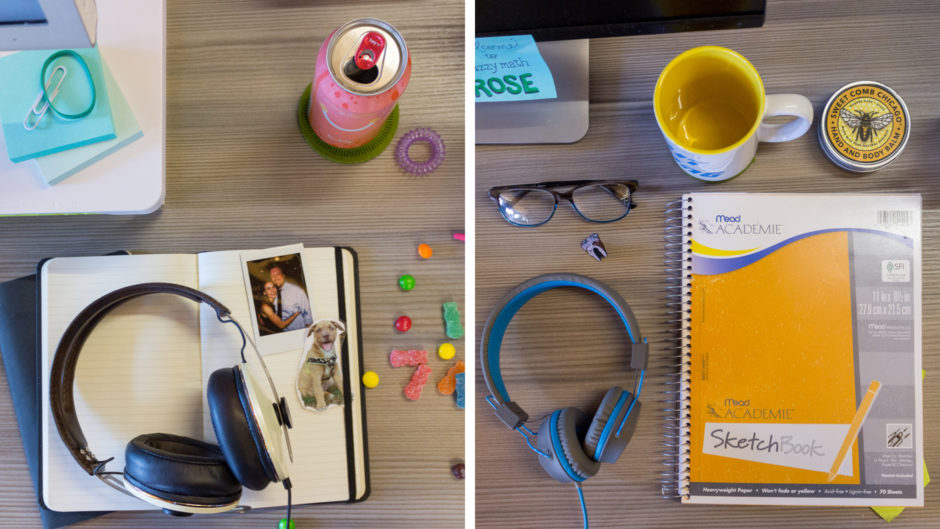Fuzzy Math Spotlight: Rachel S. & Rose

Posted by Fuzzy MathFuzzy Math Spotlight: Mia & Philip

Posted by Fuzzy MathFuzzy Math Spotlight: Kelly & Carl

Posted by Fuzzy MathFuzzy Math Spotlight: Liz & Paris

Posted by Fuzzy Math
Stay up to date on all things UX with our curated monthly newsletter.